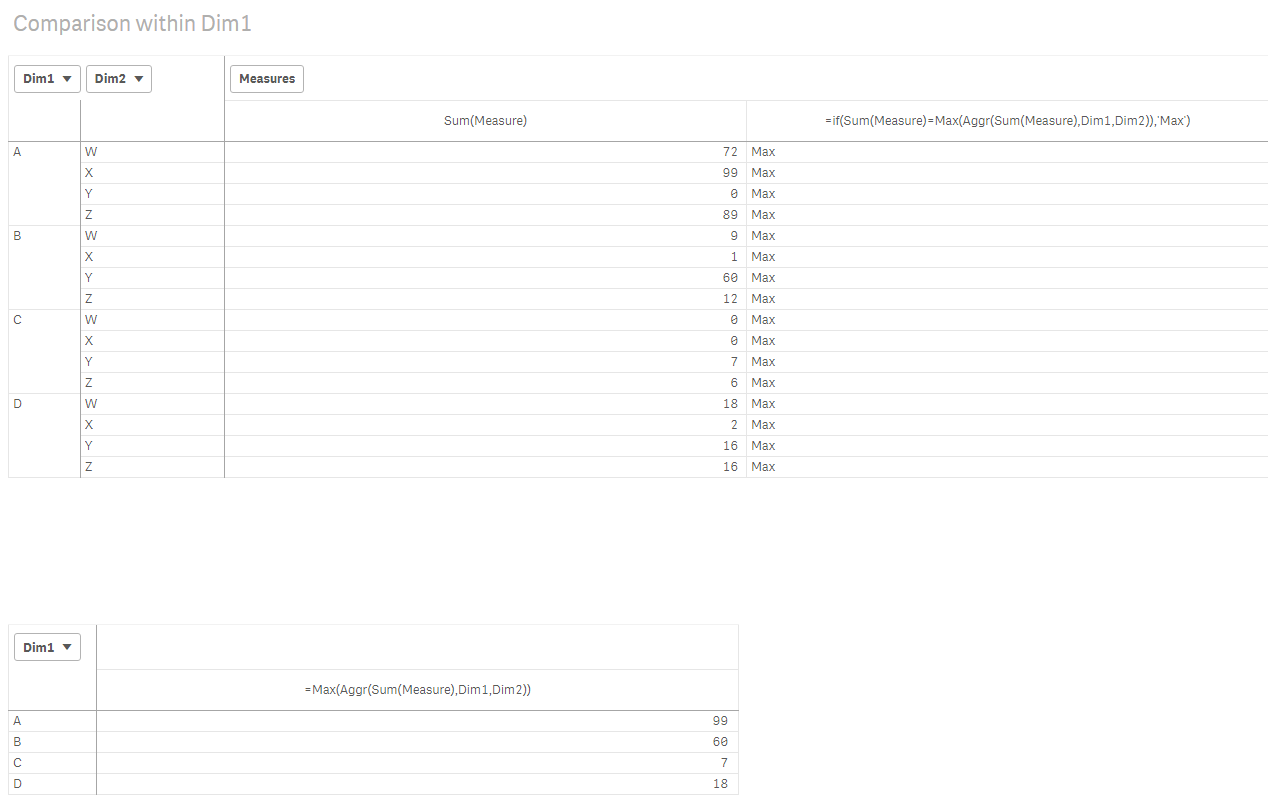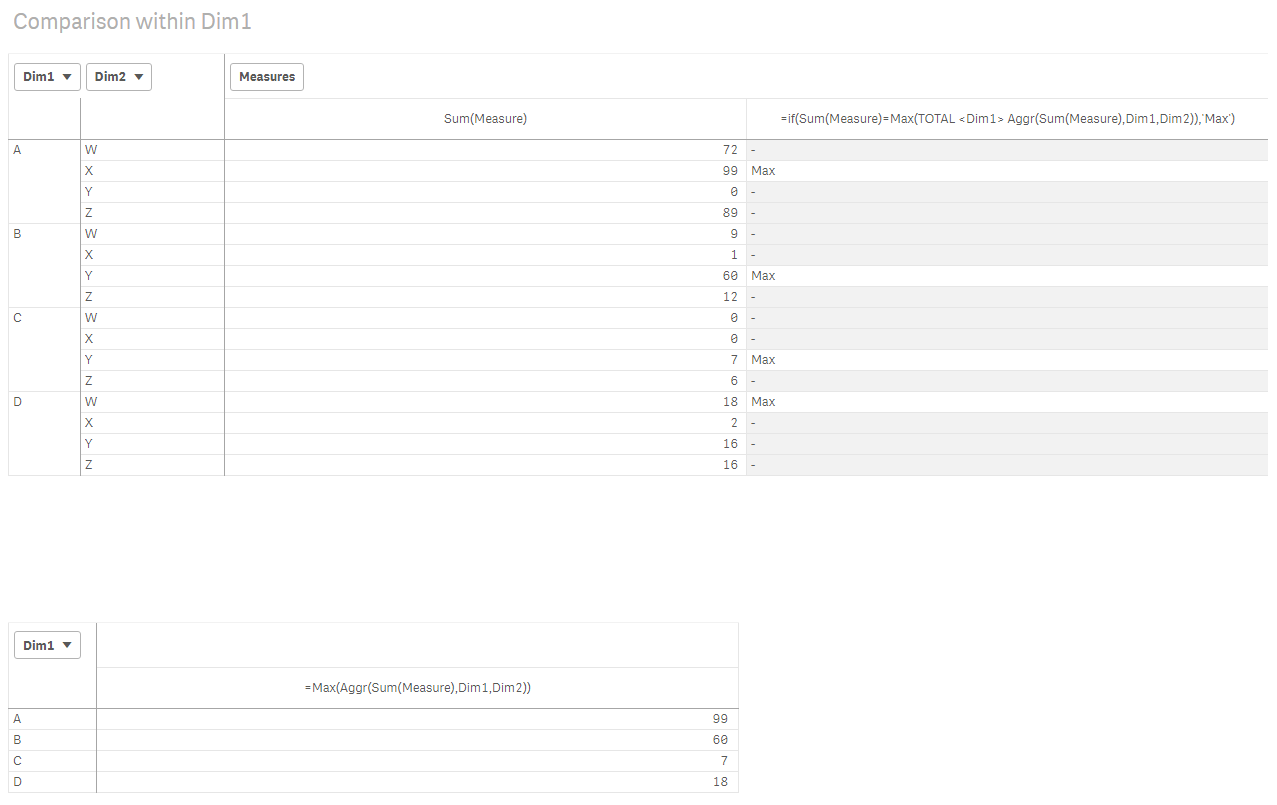# Qlik Sense App Development

Discussion board where members can learn more about Qlik Sense App Development and Usage.

Announcements
Qlik® Product Spotlight: Discover what’s possible. Get more from our products.
See for yourself. Register today.
Valued Contributor

## Finding Max

Hi there,

Brain freeze here! How do I find and mark the Max for each Dim1? How do I reduce the scope?

=if(Sum(Measure)=Max(Aggr(Sum(Measure),Dim1,Dim2)),'Max')1 Solution

Accepted SolutionsMVP

## Re: Finding Max

‌This

=if(Sum(Measure)=Max(TOTAL <Dim1>Aggr(Sum(Measure),Dim1,Dim2)),'Max')

2 RepliesMVP

## Re: Finding Max

‌This

=if(Sum(Measure)=Max(TOTAL <Dim1>Aggr(Sum(Measure),Dim1,Dim2)),'Max')

Valued Contributor

## Re: Finding Max

Thanks Sunny